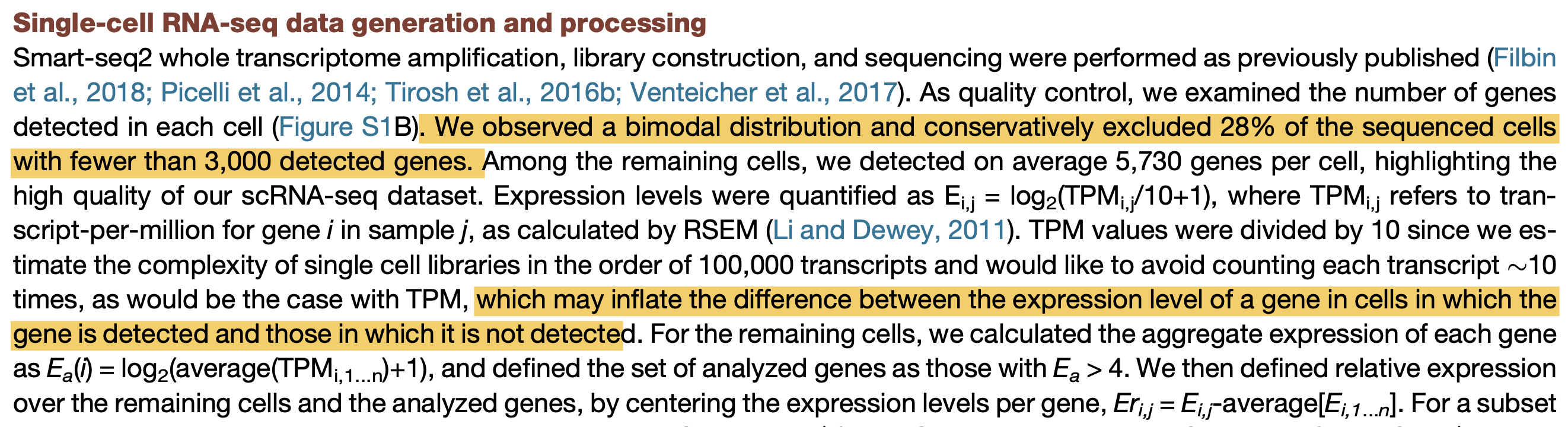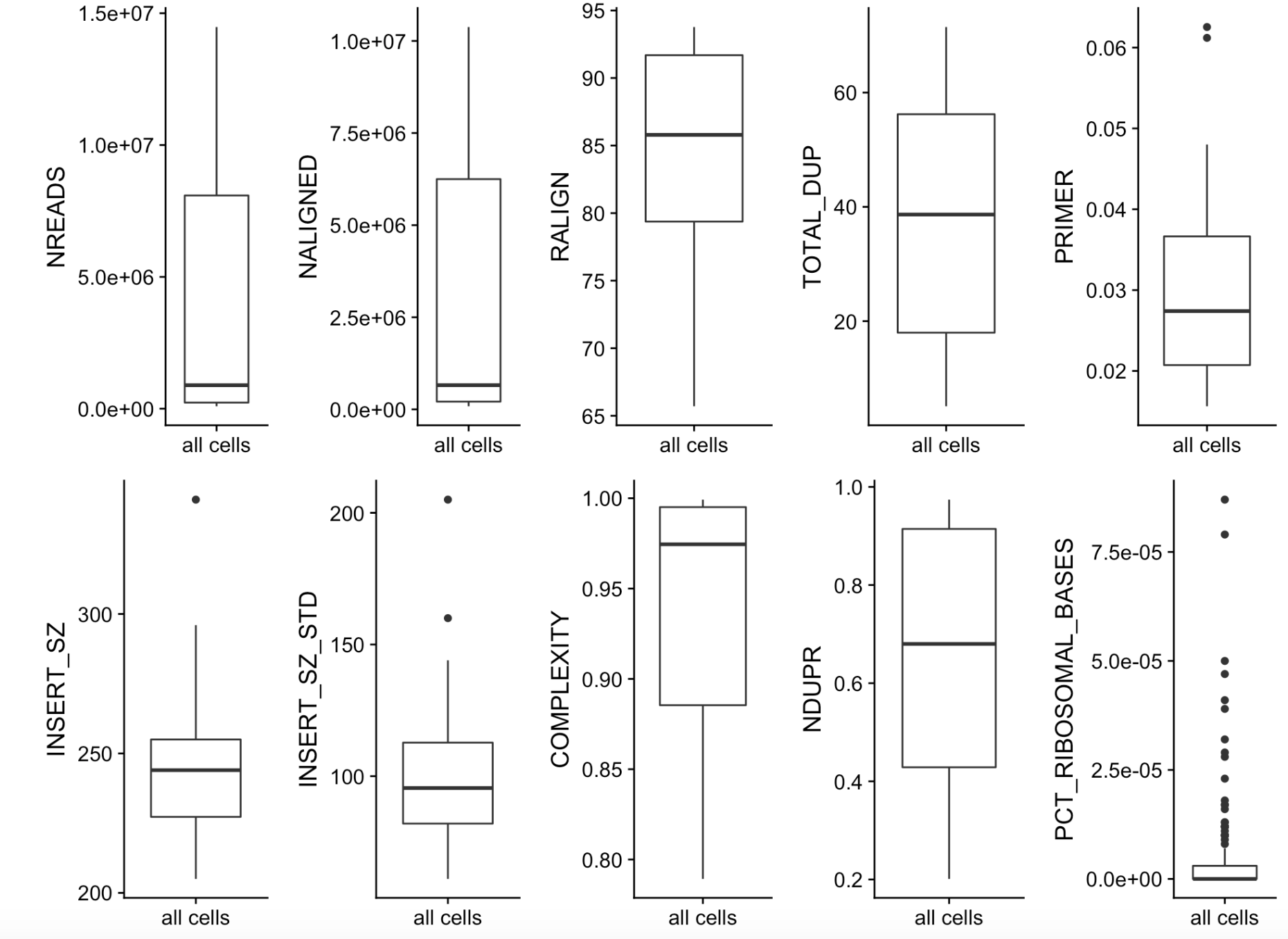# 单细胞转录组数据预处理之过滤不合格细胞和基因

### 首先细胞的取舍主要是看文库大小和检测到的基因数量``````box <- lapply(colnames(sample_ann[,1:19]),function(i) {
dat <- sample_ann[,i,drop=F]
dat\$sample=rownames(dat)
## 画boxplot
ggplot(dat, aes('all cells', get(i))) +
geom_boxplot() +
xlab(NULL)+ylab(i)
})
plot_grid(plotlist=box, ncol=5 )
``````